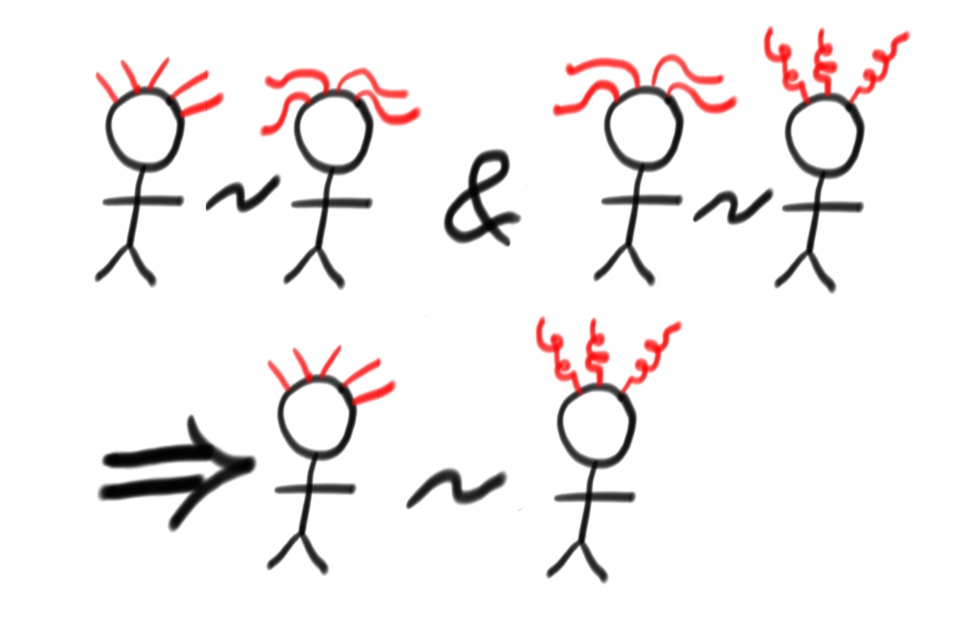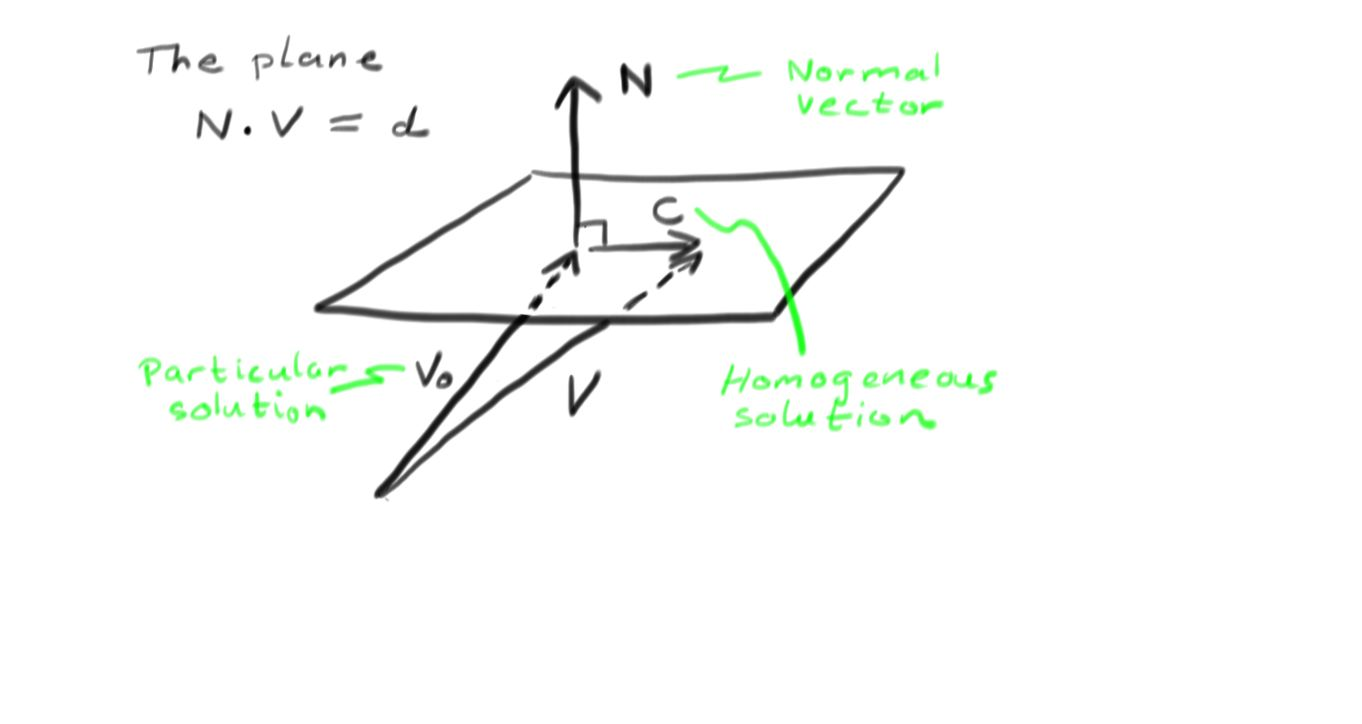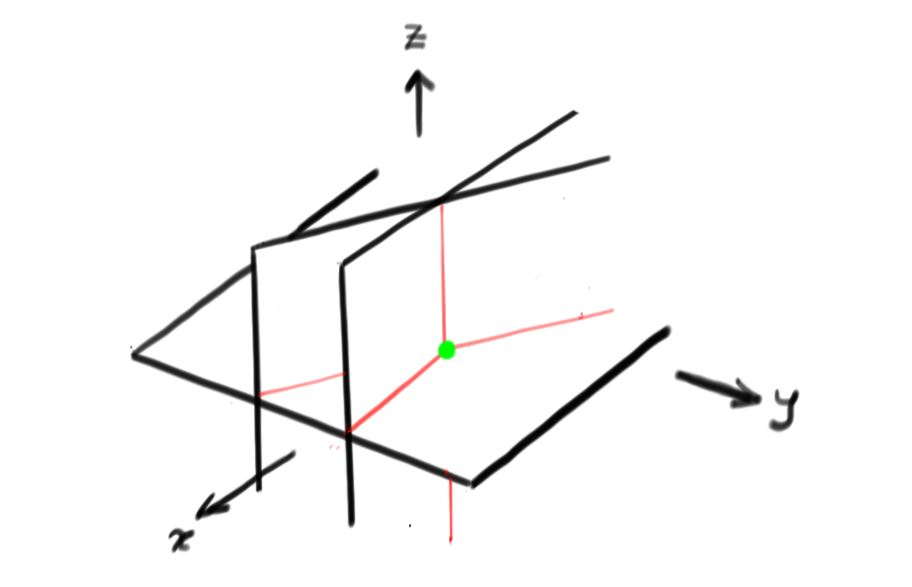$$\newcommand{\id}{\mathrm{id}}$$ $$\newcommand{\Span}{\mathrm{span}}$$ $$\newcommand{\kernel}{\mathrm{null}\,}$$ $$\newcommand{\range}{\mathrm{range}\,}$$ $$\newcommand{\RealPart}{\mathrm{Re}}$$ $$\newcommand{\ImaginaryPart}{\mathrm{Im}}$$ $$\newcommand{\Argument}{\mathrm{Arg}}$$ $$\newcommand{\norm}{\| #1 \|}$$ $$\newcommand{\inner}{\langle #1, #2 \rangle}$$ $$\newcommand{\Span}{\mathrm{span}}$$

G1: Movie Scripts 1-2

$$\newcommand{\vecs}{\overset { \rightharpoonup} {\mathbf{#1}} }$$

$$\newcommand{\vecd}{\overset{-\!-\!\rightharpoonup}{\vphantom{a}\smash {#1}}}$$

G.1: What is Linear Algebra

Hint for Review Problem 5

Looking at the problem statement we find some important information, first that oranges always have twice as much sugar as apples, and second that the information about the barrel is recorded as $$(s,f)$$, where $$s =$$ units of sugar in the barrel and $$f =$$ number of pieces of fruit in the barrel.

We are asked to find a linear transformation relating this new representation to the one in the lecture, where in the lecture $$x=$$ the number of apples and $$y=$$ the number of oranges. This means we must create a system of equations relating the variable $$x$$ and $$y$$ to the variables $$s$$ and $$f$$ in matrix form. Your answer should be the matrix that transforms one set of variables into the other.

$$\textit{Hint:}$$ Let $$\lambda$$ represent the amount of sugar in each apple.

1. To find the first equation relate $$f$$ to the variables $$x$$ and $$y$$.
2. To find the second equation, use the hint to figure out how much sugar is in $$x$$ apples, and $$y$$ oranges in terms of $$\lambda$$. Then write an equation for $$s$$ using $$x$$, $$y$$ and $$\lambda$$.

G.2: Systems of Linear Equations

Augmented Matrix Notation

Why is the augmented matrix
$$\left( \begin{array}{cc | c} 1 & 1 & 27 \\ 2 & -1 & 0 \end{array} \right)\, ,$$
equivalent to the system of equations
\begin{eqnarray*}
x+y &=& 27\\
2x - y &=& 0\, ?
\end{eqnarray*}
Well the augmented matrix is just a new notation for the matrix equation
\begin{equation*}
\begin{pmatrix}
1 &1 \\
2 &-1
\end{pmatrix}
\begin{pmatrix}x \\ y\end{pmatrix}
=
\begin{pmatrix}27 \\ 0\end{pmatrix}
\end{equation*}
and if you review your matrix multiplication remember that
\begin{equation*}
\begin{pmatrix}
1 &1 \\
2 &-1
\end{pmatrix}
\begin{pmatrix}x \\ y\end{pmatrix}
=
\begin{pmatrix}x+ y \\ 2x - y\end{pmatrix}
\end{equation*}
This means that

\begin{equation*}
\begin{pmatrix}x+ y \\ 2x - y\end{pmatrix}
=
\begin{pmatrix}27 \\ 0\end{pmatrix}\, ,
\end{equation*}
which is our original equation.

Equivalence of Augmented Matrices

Lets think about what it means for the two augmented matrices
$$\left( \begin{array}{cc | c} 1 & 1 & 27 \\ 2 & -1 & 0 \end{array} \right) \mbox{ and } \left( \begin{array}{cc | c} 1 & 0 & 9 \\ 0 & 1 & 18 \end{array} \right)$$
to be equivalent:
They are certainly not equal, because they don't match in each component, but since these augmented matrices represent a system, we might want to introduce a new kind of equivalence relation.

Well we could look at the system of linear equations this represents

\begin{eqnarray*}
x+y &=& 27\\
2x - y &=& 0\,
\end{eqnarray*}
and notice that the solution is $$x=9$$ and $$y=18$$. The other augmented matrix represents the system
\begin{eqnarray*}
x\ +0 \cdot y &=& 9\\
0 \cdot x \ +\ \phantom{0 \cdot} y &=& 18\,
\end{eqnarray*}
This clearly has the same solution. The first and second system are related in the sense that their solutions are the same. Notice that it is really nice to have the augmented matrix in the second form, because the matrix multiplication can be done in your head.

Hints for Review Question 10

This question looks harder than it actually is: Row equivalence of matrices is an example of an $$\textit{equivalence relation}$$. Recall that a relation $$\sim$$ on a set of objects $$U$$ is an equivalence relation if the following three properties are satisfied:

• Reflexive: For any $$x\in U$$, we have $$x\sim x$$.
• Symmetric: For any $$x,y \in U$$, if $$x\sim y$$ then $$y\sim x$$.
• Transitive: For any $$x,y$$ and $$z \in U$$, if $$x\sim y$$ and $$y\sim z$$ then $$x\sim z$$.

Show that row equivalence of augmented matrices is an equivalence relation.

Firstly remember that an equivalence relation is just a more general version of "equals''. Here we defined row equivalence for augmented matrices whose linear systems have solutions by the property that their solutions are the same.

So this question is really about the word {\it same}. Lets do a silly example: Lets replace the set of augmented matrices by the set of people who have hair. We will call two people equivalent if they have the same hair color. There are three properties to check:

• Reflexive: This just requires that you have the same hair color as yourself so obviously holds.• Symmetric: If the first person, Bob (say) has the same hair color as a second person Betty(say), then Bob has the same hair color as Betty, so this holds too.• Transitive: If Bob has the same hair color as Betty (say) and Betty has the same color as Brenda (say), then it follows that Bob and Brenda have the same hair color, so the transitive property holds too and we are done.Solution Set in Set Notation

Here is an augmented matrix, let's think about what the solution set looks like
$$\left( \begin{array}{ccc | c} 1 & 0 & 3 & 2 \\ 0 & 1 & 0 & 1 \end{array} \right)$$
This looks like the system
\begin{eqnarray*}
1\cdot x_{1}\phantom{+x_{2}}\ + \ 3x_{3} &=& 2\\
1\cdot x_{2}\phantom{\ +\ 3x_{3} } &=& 1
\end{eqnarray*}
Notice that when the system is written this way the copy of the $$2 \times 2$$ identity matrix $$\begin{pmatrix}1 & 0\\ 0 & 1\end{pmatrix}$$ makes it easy to write a solution in terms of the variables $$x_{1}$$ and $$x_{2}$$. We will call $$x_{1}$$ and $$x_{2}$$ the $$\textit{pivot}$$ variables. The third column $$\begin{pmatrix}3 \\0\end{pmatrix}$$ does not look like part of an identity matrix, and there is no $$3\times 3$$ identity in the augmented matrix. Notice there are more variables than equations and that this means we will have to write the solutions for the system in terms of the variable $$x_{3}$$. We'll call $$x_{3}$$ the $$\textit{free}$$ variable.

Let $$x_{3} = \mu$$. (We could also just add a "dummy'' equation $$x_{3}=x_{3}$$.) Then we can rewrite the first equation in our system
\begin{eqnarray*}
x_1+ 3x_3 &=& 2\\
x_1+ 3\mu &=& 2\\
x_1 &=& 2 -3\mu.
\end{eqnarray*}
Then since the second equation doesn't depend on $$\mu$$ we can keep the equation
$$x_{2} = 1,$$
and for a third equation we can write
$$x_{3} = \mu$$
so that we get the system
\begin{eqnarray*}
\begin{pmatrix}x_{1} \\ x_{2} \\ x_{3}\end{pmatrix} &=& \begin{pmatrix}2 - 3\mu \\ 1 \\ \mu\end{pmatrix}\\
&=& \begin{pmatrix}2 \\ 1 \\ 0\end{pmatrix} + \begin{pmatrix}- 3\mu \\ 0 \\ \mu\end{pmatrix} \\
&=& \begin{pmatrix}2 \\ 1 \\ 0\end{pmatrix} + \mu \begin{pmatrix}- 3 \\ 0 \\ 1\end{pmatrix}.
\end{eqnarray*}
Any value of $$\mu$$ will give a solution of the system, and any system can be written in this form for some value of $$\mu$$. Since there are multiple solutions, we can also express them as a set:
$\left\{ \begin{pmatrix}x_{1} \\ x_{2} \\ x_{3}\end{pmatrix} = \begin{pmatrix}2 \\ 1 \\ 0\end{pmatrix} + \mu \begin{pmatrix}- 3 \\ 0 \\ 1\end{pmatrix} \; \mid \; \mu \in \mathbb{R} \right\}.$

Worked Examples of Gaussian Elimination

Let us consider that we are given two systems of equations that give rise to the following two (augmented) matrices:
$$\left(\begin{array}{rrrr|r}2&5&2&0&2\\1&1&1&0&1\\1&4&1&0&1\end{array}\right) \quad \quad \left(\begin{array}{rr|r}5&2&9\\0&5&10\\0&3&6\end{array}\right)$$
and we want to find the solution to those systems. We will do so by doing Gaussian elimination.

For the first matrix we have
\begin{align*}\left(\begin{array}{rrrr|r}
2 & 5 & 2 & 0 & 2 \\
1 & 1 & 1 & 0 & 1 \\
1 & 4 & 1 & 0 & 1
\end{array}\right)
\overset{R_{1} \leftrightarrow R_{2}}{\sim} &
\left(\begin{array}{rrrr|r}
1 & 1 & 1 & 0 & 1 \\
2 & 5 & 2 & 0 & 2 \\
1 & 4 & 1 & 0 & 1
\end{array}\right)
\\ \overset{R_{2} - 2 R_{1} ; R_{3} - R_{1}}{\sim} &
\left(\begin{array}{rrrr|r}
1 & 1 & 1 & 0 & 1 \\
0 & 3 & 0 & 0 & 0 \\
0 & 3 & 0 & 0 & 0
\end{array}\right)
\\ \overset{\frac{1}{3}R_{2}}{\sim} &
\left(\begin{array}{rrrr|r}
1 & 1 & 1 & 0 & 1 \\
0 & 1 & 0 & 0 & 0 \\
0 & 3 & 0 & 0 & 0
\end{array}\right)
\\ \overset{R_{1} - R_{2} ; R_{3} - 3 R_{2}}{\sim} &
\left(\begin{array}{rrrr|r}
1 & 0 & 1 & 0 & 1 \\
0 & 1 & 0 & 0 & 0 \\
0 & 0 & 0 & 0 & 0
\end{array}\right)\end{align*}

1. We begin by interchanging the first two rows in order to get a 1 in the upper-left hand corner and avoiding dealing with fractions.
2. Next we subtract row 1 from row 3 and twice from row 2 to get zeros in the left-most column.
3. Then we scale row 2 to have a 1 in the eventual pivot.
4. Finally we subtract row 2 from row 1 and three times from row 2 to get it into Reduced Row Echelon Form.

Therefore we can write $$x = 1 - \lambda$$, $$y = 0$$, $$z = \lambda$$ and $$w = \mu$$, or in vector form
$\begin{pmatrix}x\\y\\z\\w\end{pmatrix} = \begin{pmatrix}1\\0\\0\\0\end{pmatrix} + \lambda \begin{pmatrix}-1\\0\\1\\0\end{pmatrix} + \mu \begin{pmatrix}0\\0\\0\\1\end{pmatrix}.$

Now for the second system we have
\begin{align*}
\left(\begin{array}{rr|r}
5 & 2 & 9 \\
0 & 5 & 10 \\
0 & 3 & 6
\end{array}\right)
\overset{\frac{1}{5}R_{2}}{\sim} &
\left(\begin{array}{rr|r}
5 & 2 & 9 \\
0 & 1 & 2 \\
0 & 3 & 6
\end{array}\right)
\\ \overset{R_{3} - 3 R_{2}}{\sim} &
\left(\begin{array}{rr|r}
5 & 2 & 9 \\
0 & 1 & 2 \\
0 & 0 & 0
\end{array}\right)
\\ \overset{R_{1} - 2 R_{2}}{\sim} &
\left(\begin{array}{rr|r}
5 & 0 & 5 \\
0 & 1 & 2 \\
0 & 0 & 0
\end{array}\right)
\\ \overset{\frac{1}{5}R_{1}}{\sim} &
\left(\begin{array}{rr|r}
1 & 0 & 1 \\
0 & 1 & 2 \\
0 & 0 & 0
\end{array}\right)
\end{align*}
We scale the second and third rows appropriately in order to avoid fractions, then subtract the corresponding rows as before. Finally scale the first row and hence we have $$x = 1$$ and $$y = 2$$ as a unique solution.

Planes

Here we want to describe the mathematics of planes in space. The video is summarised by the following picture:A plane is often called $$\mathbb{R}^{2}$$ because it is spanned by two coordinates, and space is called $$\mathbb{R}^{3}$$ and has three coordinates, usually called $$(x,y,z)$$. The equation for a plane is
$$ax+by+cz=d\, .$$
Lets simplify this by calling $$V=(x,y,z)$$ the vector of unknowns and $$N=(a,b,c)$$. Using the dot product in $$\mathbb{R}^{3}$$ we have
$$N\cdot V = d\, .$$
Remember that when vectors are perpendicular their dot products vanish. $$\textit{I.e.} \(U\cdot V = 0 \Leftrightarrow U \perp V$$. This means that if a vector $$V_{0}$$ solves our equation $$N\cdot V =d$$, then so too does $$V_{0}+C$$ whenever $$C$$ is perpendicular to $$N$$. This is because
$$N\cdot (V_{0}+C) = N\cdot V_{0} + N\cdot C = d + 0 = d\, .$$
But $$C$$ is ANY vector perpendicular to $$N$$, so all the possibilities for $$C$$ span a plane whose normal vector is $$N$$. Hence we have shown that solutions to the equation $$ax+by+cz=0$$ are a plane with normal vector $$N=(a,b,c)$$.

Pictures and Explanation

This video considers solutions sets for linear systems with three unknowns. These are often called $$(x,y,z)$$ and label points in $$\mathbb{R}^{3}$$. Lets work case by case:

• If you have no equations at all, then any $$(x,y,z)$$ is a solution, so the solution set is all of $$\mathbb{R}^{3}$$. The picture looks a little silly:• For a single equation, the solution is a plane. This is explained in this video. The picture looks like this:• For two equations, we must look at two planes. These usually intersect along a line, so the solution set will also (usually) be a line:• For three equations, most often their intersection will be a single point so the solution will then be unique:• Of course stuff can go wrong. Two different looking equations could determine the same plane, or worse equations could be inconsistent. If the equations are inconsistent, there will be no solutions at all. For example, if you had four equations determining four parallel planes the solution set would be empty. This looks like this: